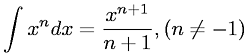Equations > Calculus > Integration/Integrals > Integral of powers not equal to -1

### Integral of powers not equal to -1Latex Code:

MathML Code:

 $\int {x}^{n}\mathrm{dx}=\frac{x}^{n+1}n+1,\left(n\ne -1\right)$

MathType 5.0: